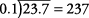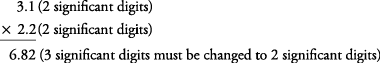## Significant Digits

When a digit tells how many units of measure are involved, it is a significant digit. To find the number of units of measure, simply divide the actual measurement by the unit of measure.

##### Example 1

Find the number of units used and the significant digits for each of the following.

1. 23.7 m (unit of measure, 0.1 m)

2. 0.05 cm (unit of measure, 0.01 cm)

3. 520 g (unit of measure, 1 g)

4. 760 km (unit of measure, 10 km)

5. 2100 m (unit of measure, 10 m)

6. 2100 m (unit of measure, 100 m)

For each of these examples, find the number of units of measure by dividing the actual measurement by the unit of measure. For example, for (a),The following rules can be used as general guidelines for determining significant digits:

• Digits that are not zero are always significant. For example, all three digits are significant in 35.7.
• Zeros at the end (or right) of the decimal are always significant. For example, both digits are significant in .20.
• In a decimal, zeros in front (or to the left) of a significant digit are never significant. For example, only 7 is significant in .007.
• All zeros that are between significant digits are significant. For example, all digits are significant in 500.5.
• Depending on the unit of measure, zeros that are at the end (or right) of a whole number may or may not be significant. For example, 500 m measured to the 1 m gives three significant digits (5, 0, and 0), whereas 500 m measured to the 10 m gives two significant digits (5 and 0).

When computing using significant digits, you should always round the answer to the smallest number of significant digits in any of the numbers being used.

##### Example 2

Find the area of the rectangle with length 3.1 m and width 2.2 m.So 6.82 ≈ 6.8 sq m.

##### Example 3

Find the length of a rectangle with area 19 sq cm and width 4 cm.

Because 19 has two significant digits and 4 has one significant digit, the answer must be rounded to one significant digit.So 4.75 ≈ 5 cm.